# Definition: Laplacian Matrix for triangle meshesQuick definition of the Laplacian matrix on a triangular mesh. I give an in depth explanation here.

# [Maya C++ API] paint weights with MPxDeformer# Distributing Maya pluginsI will discuss various ways you can ship a Maya plugin to users.

# Polar decomposition of a 3x3 matrixLeaving [C++ code ] to decompose a 3x3 matrix into rotation and scale/shear with polar decomposition (this is done through a 3x3 SVD and 3x3 Eigen decomposition).

Keywords:

• Polar decomposition of a 3x3 matrix
• 3x3 matrix SVD (Singular Value decomposition)
• Eigen decomposition (extract eigenvalues and eigenvectors) of a 3x3 matrix M (assumes symmetric matrix)

# Diffusing / smoothing weight map over a triangular mesh.Showcasing simple procedures with C++ code to smooth / diffuse per vertex weights over a triangle mesh.

# Inspiration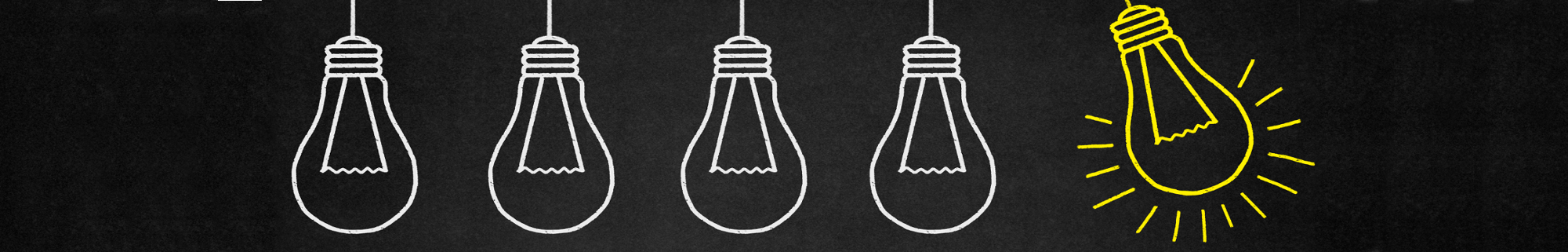# Normal to an implicit surface# Implicit surface a.k.a (signed) distance field: definition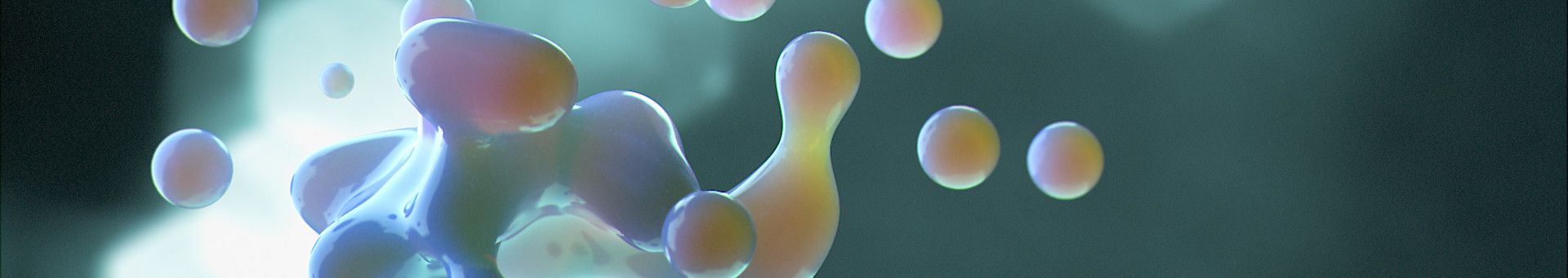If I say "implicit surface" you may think of metaballs and marching cube, I'll show you there is more to it!

# Phong illumination model (cheat sheet)

$$f( \vec p ) = \underbrace{I_a K_a}_{\color{red} \text{ambient component}} + \underbrace{\sum_i^\text{nb_lights} (\vec n( \vec p ) . \vec l_i) K_d I_i}_{\color{green} \text{diffuse component}} + \underbrace{\sum_i^\text{nb_lights} f_{spec}(\vec l_i( \vec p ), \vec v(\vec p)) K_s I_i}_{\color{blue} \text{specular component}}$$

I summarize the Phong illumination model with the above equation and explain all the terms one by one.

# Anki tip, inserting furigana (pop up on mouse hover)Here is how you can make furigana appear above kanjis in your anki flash cards like this: おはし上手じょうずですね！

# Learning japanese: a life's journey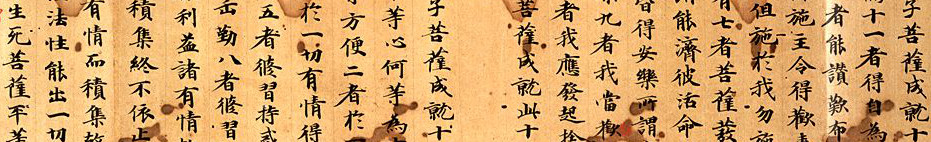Learning a language is quite a challenge, here I will gather some resources and thoughts about learning Japanese.

# MAYA_SHELF_PATH not working, empty Maya shelves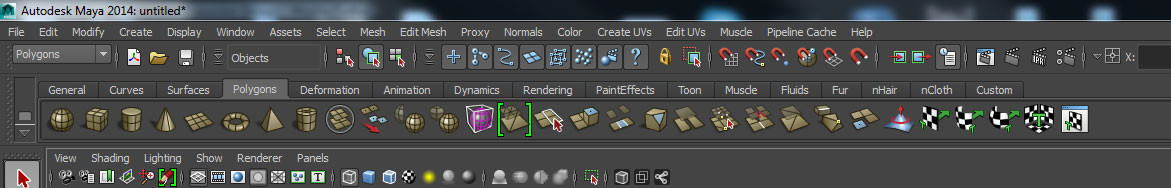# [Maya C++ API] Get selected vertices of a mesh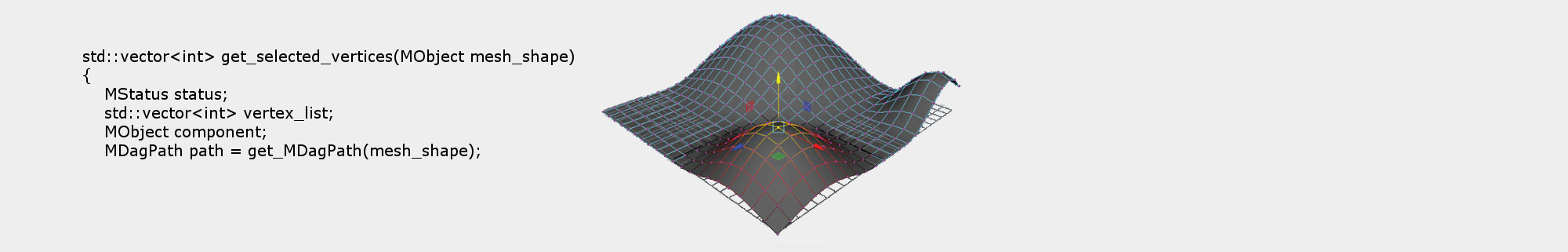# [Maya C++ API] Set skinning weight attributes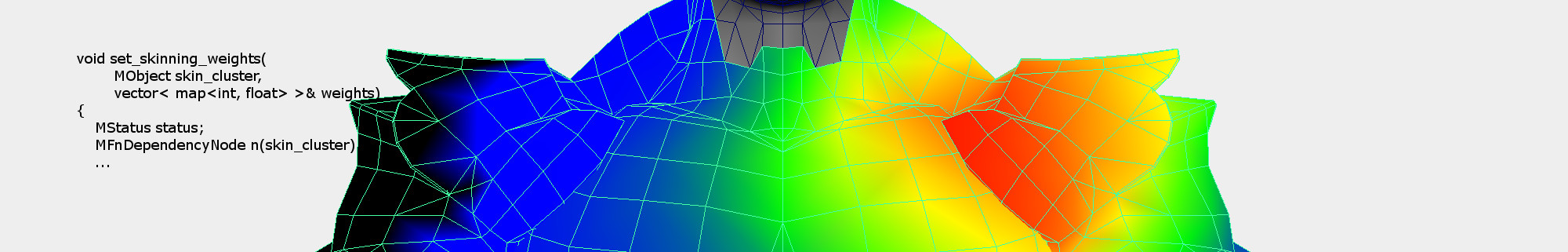Some C++ Maya API code to set skin weights (multi attributes) of a skin cluster node.

# Dual Quaternion Skinning with scale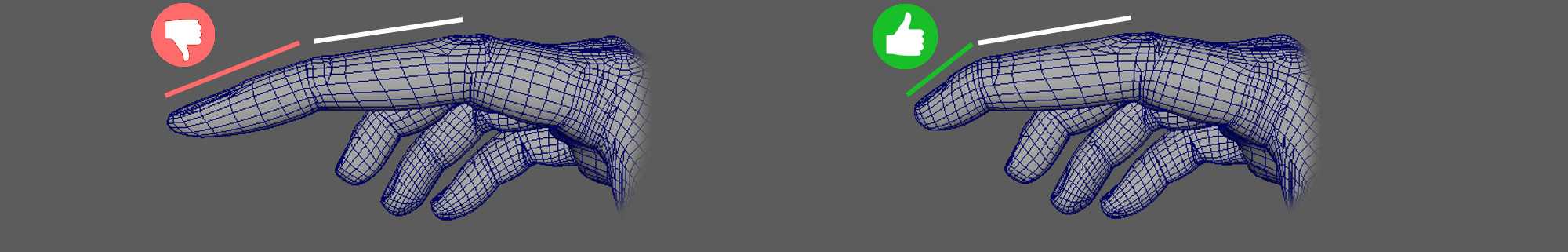DQS with scale applied on the second to last joint. Left, globally propagates until the last bone, right, scale localized to each joint.

# Skeletal animation, forward kinematic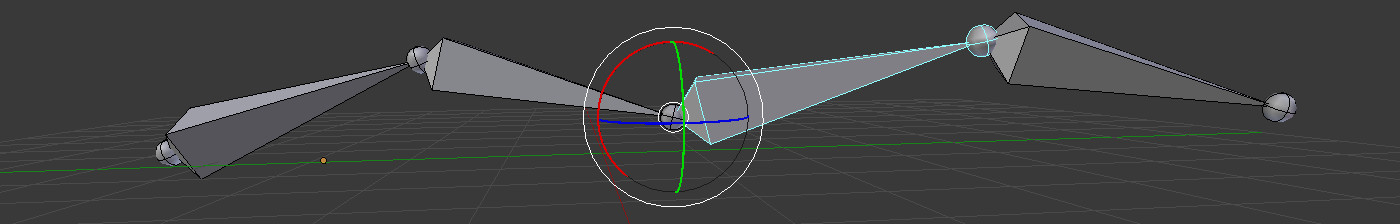# Maya MEL commands and procedures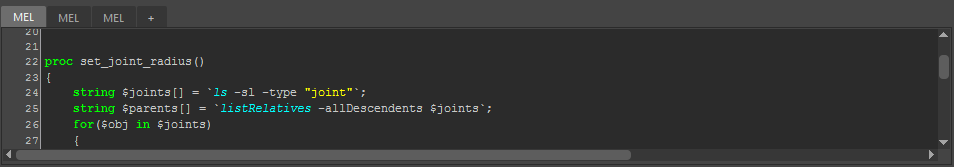# Transforming implicit surface (distance field) and their gradient# [Maya C++ API] Save MPxData attribute on file# [Maya C++ API] Unable to unload plugin in Maya# Bulge free Dual Quaternion Skinning (Trick)C++ code snippet to correct the Dual Quaternion Skinning bulge artifact. Left: standard DQS, right bulge correction, (automatic skin weight with Maya "smooth bind").

# [Maya C++ API] Per vertex color update in MPxDeformerNodeCode snippet in C++ to be able to update the color of a mesh with Maya API on a per vertex basis.

# MAYA MEL wait for idle events/evalDeferred to finish

A trick to pause the script until evalDeferred / scriptJob / idle are executed.

# Laplacian smoothing (C++ code to smooth a mesh)Dropping a procedure to compute the Laplacian smoothing of a 3D mesh (with cotangent weights).

# C++ code for cotangent weights over a triangular mesh$$w_{ij} = \cot(\alpha_{ij}) + \cot(\beta_{ij})$$

# Implicit skinning routines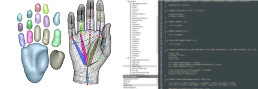# Transform normals given a deformation map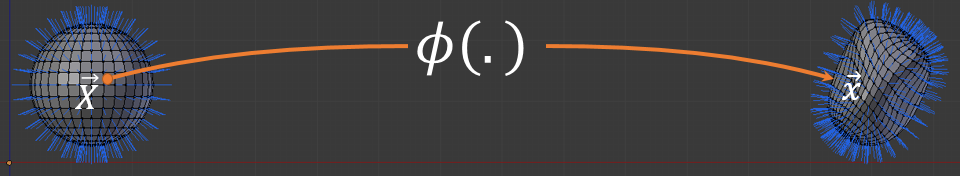Changing the shape of a 3D mesh is a very common operation in computer graphics. For instance, rotations, translations etc. are very basic forms of mesh deformations. Of course more complicated algorithms exists to deform your mesh such as wrap deformers, linear blending skinning and so on... During the design of these algorithms you might face a question: how do I transform the normal of the original shape in rest pose to the deformed shape? This entry will give you some pointers to compute it.

# Blender tutorial to animate a cylinder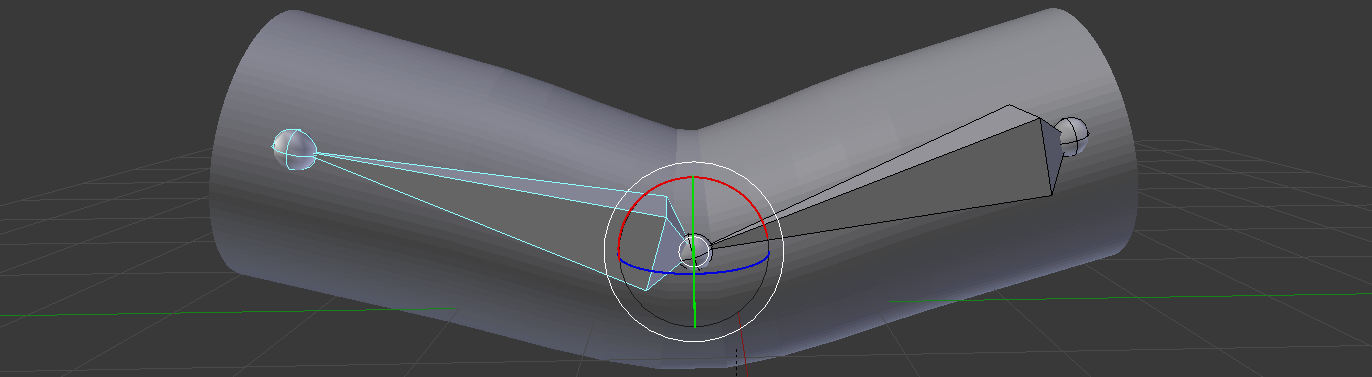The goal of this tutorial is to describe step by step the process of skinning a simple cylinder with two joints in Blender 2.5 and superior. This is a beginner lesson in the sense that I will describe as much as possible shortcuts and actions, however, there will be no high level explanations.

Note for the scholars: blender implements the heat diffusion method to compute skinning weights, linear blending and dual quaternion skinning are used to deform the mesh.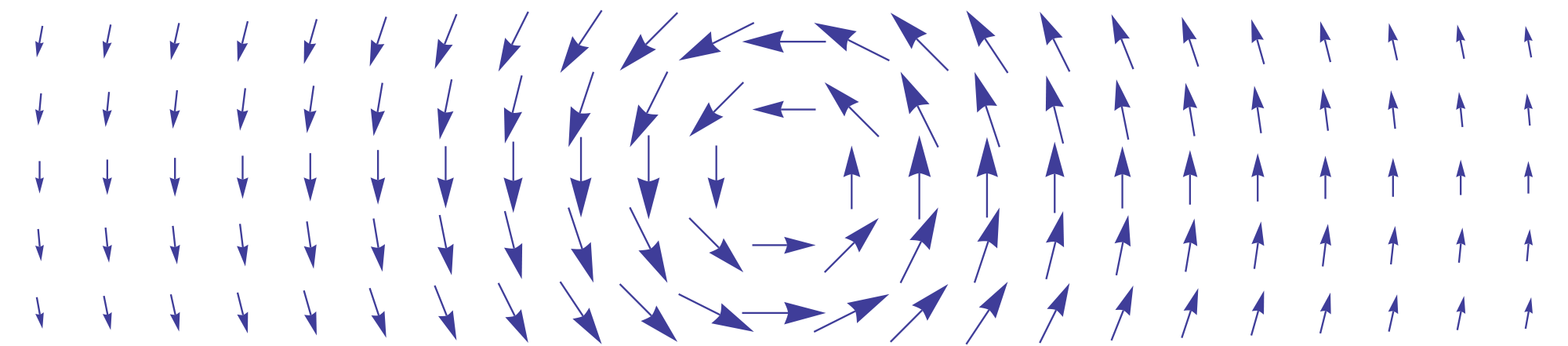Just leaving some notes to differentiate expressions with the $$\nabla$$ operator to compute gradients of various functions.

Here you will find our Implicit skinning standalone application under the license GNU GPL3.

Disclaimer
The standalone application is written in Qt/C++ and CUDA, therefore it only works on NVIDIA GPUs but should run on Windows or Linux. This code is a research project developed on three Linux machines with similar configurations and "tested" by very few people: expect difficulties to get it to work. The documentation is also very thin. That being said I will be happy to answer questions in case of troubles.

# Elastic Implicit Skinning: a Robust Iso-Surface Tracking for Interactive Character Skinning

Publication: ACM SIGGRAPH ASIA, 2014

#### Rodolphe Vaillant1,2, Gaël Guennebaud3, Loïc Barthe1, Brian Wyvill2, Marie-Paule Cani4

1IRIT - Université de Toulouse, 2University of Victoria, 3Inria Bordeaux,
4LJK - Grenoble Universités - InriaComparison of Implicit Skinning with our new Elastic Implicit Skinning. From right to left: Jeff in T-pose, Jeff rigged with implicit skinning, Jeff rigged with elastic implicit skinning, female model with implicit skinning then elastic implicit skinning.

With elastic implicit skinning the skin stretches automatically (without skinning weights) and the vertices distribution is more pleasing (notice the belly button stretch). Our approach is more robust, for instance the angle's range of joints is larger than implicit skinning (notice the triangle inversion of implicit skinning at the knee joint)

# 2D biharmonic stencil a.k.a bilaplacian operator

Draft work in progress

# Voro++.0.4.5 with cmake for easy compilation under windows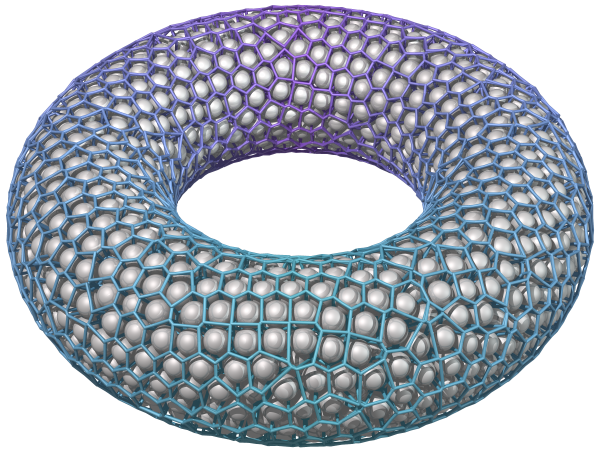Dropping my code of the [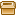cmake version of voro++0.4.5 ]. It helped me to compile the voro++ library under windows with cmake.

# Harmonic function: definitions and propertiesThis is the second part of my tutorial series on bounded harmonic functions. For a quick introduction and examples of use of harmonic functions read the first part. In this part I define harmonic functions and their properties. This is the hard part with a lot of mathematics. But it's a mandatory step to understand how harmonic functions work. This will allow you to apply them in a broader context and understand many scientific papers relying on these. In addition this will be my entry point to introduce Finite Element Method in future posts. So hang on it's worth it!

# AnkiDroid: How to solve "Syncing error, type: 409, message: Conflict" problem

I ran into the infamous message "Syncing error, type: 409, message: Conflict" while syncing my AnkiDroid with AnkiWeb on my Nexus 4. Here is the solution:

# Contour lines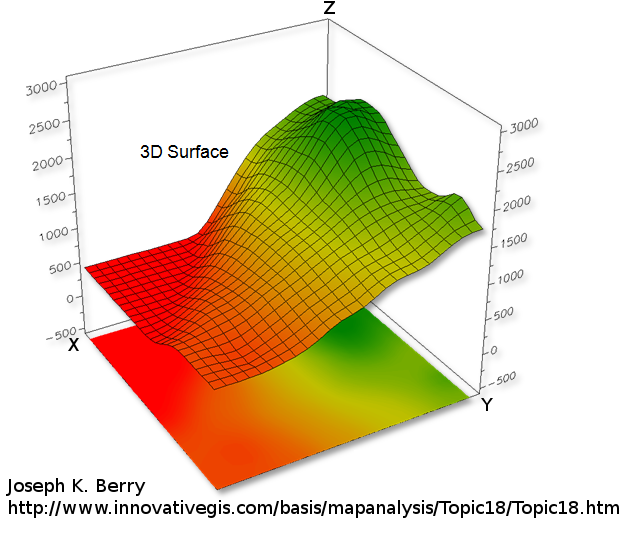## Introduction

To represent a 2D function $$z = f(x,y)$$ one can draw a 3D surface. The higher the $$z$$ value the higher the point on the surface (see above).

# How to generate bounded harmonic weights on a regular grid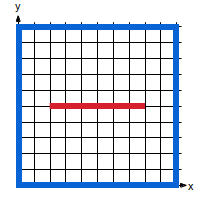(a)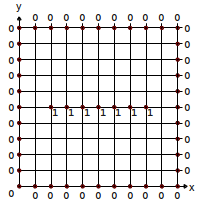(b)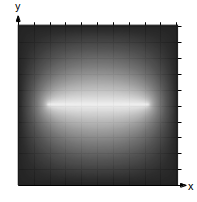(c)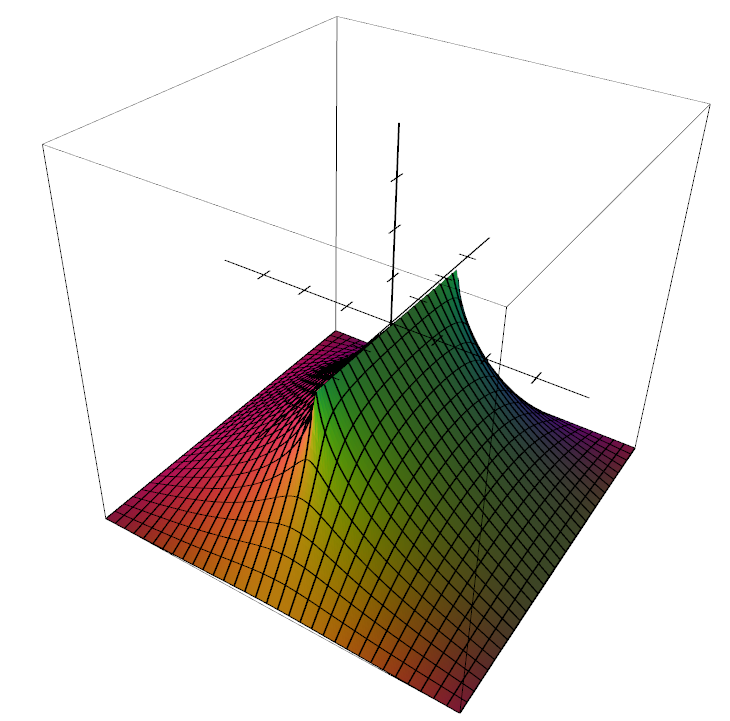(d)
 Generating an height-map  is a good example to demonstrate how useful harmonic functions can be. In (a) user can stroke lines corresponding to different heights. In (b) we associate a height where the strokes lies. In (c) we compute the rest of the values automatically (black is zero, white is one). (d) is a 3D view of the heightmap in (c). It is a 2D function $$f: \mathbb R^2 \rightarrow \mathbb R$$ which returns the height. The tutorial explains how to compute the unknown heights and ensure $$f$$ is an harmonic function.

## Summary

In this tutorial I give the basics to be able to compute harmonic functions $$f: \mathbb R^n \rightarrow \mathbb R$$ over a regular grid/texture (in 1D, 2D or 3D). All you need is to set a few values on the grid that define a closed region (see figure above), then the remaining values are automatically computed using the so-called Laplace operator and solving a linear system of equations. After a quick and "mathless" presentation of an easy way to implement this in C++, I will explain the mathematical jargon. This tutorial is the first of series which will lead to more complicated techniques like Finite Element Methods (FEM).

# Singular value decomposition of a 2x2 matrix (C++ code)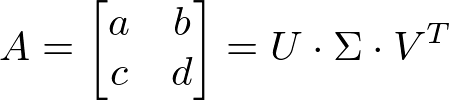Dropping some [code here] to do a singular value decomposition (SVD) of a 2 by 2 real matrix.

# Source code for poisson disk sampling of a triangle mesh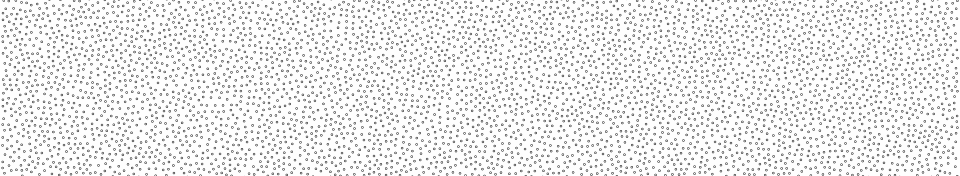Here you candownload the C++ code] to do a Poisson disk sampling of a triangular mesh. The code is a simple wrapper for the vcglib library. It takes in input a std::vector of triangles and vertex position/normals and outputs std::vector of samples positions/normals computed with the Poisson disk sampling.

EDIT: Victor Martins pointed out that the above code doesn't compile on MAC and kindly provided an [update] bundled with a newer version of vcglib.

N.B: if you are looking for a way to do Poisson disk sampling fast/in real-time take a look at this siggraph paper and matlab code. There is also a C++ implementation here.

# 3D viewer for Hermite Radial Basis Function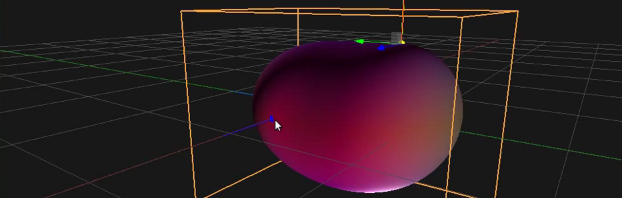This is a simple demonstrator of the HRBF technique presented and explained here. You can visualize and edit the implicit surface generated with HRBF.

# C++ code for spline curves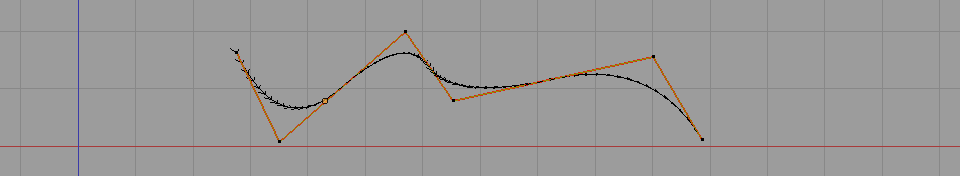Dropping on github C++ code to compute spline curves of arbitrary dimensions 3D curves or 2D splines anything is possible. The class can be instantiated with any point type (1d (float), 2d, 3d etc.) as long as the appropriate operator overload are implemented. This class use the efficient blossom algorithm to compute a position on the curve.

# Implicit Skinning: Real-Time Skin Deformation with Contact Modeling

Publication: ACM SIGGRAPH, 2013

#### Rodolphe Vaillant1,2, Loïc Barthe1, Gaël Guennebaud3, Marie-Paule Cani4, Damien Rhomer5, Brian Wyvill2, Olivier Gourmel1 and Mathias Paulin1

1IRIT - Université de Toulouse, 2University of Victoria, 3Inria Bordeaux,
4LJK - Grenoble Universités - Inria, 5CPE Lyon - Inria(e) (a) (b)(c) (d) (a) Self-intersection of the Armadillo's knee with dual quaternions skinning. (b) Implicit Skinning produces the skin fold and contact. (c), (d) two poses of the finger with Implicit Skinning generating the contact surface and organic bugle. (e) Implicit Skinning of an animated character around 95fps.

# Dual Quaternions skinning tutorial and C++ codes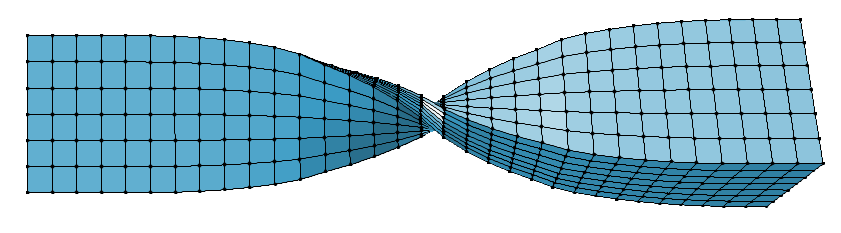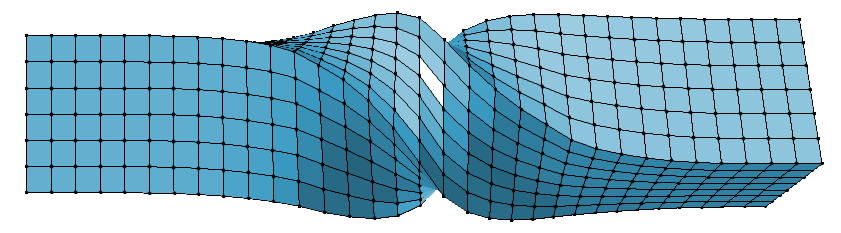In this entry I provide [C++ codes ] to deform a mesh with the famous Dual Quaternion Skinning (DQS) deformer.

# Convert implicit surface defined with global support to compact supportIn mathematics functions are sometime characterized as compactly or globally supported. I will explain what it means and how to convert a function from global to compact support in the context of implicit surface modeling (i.e. boolean modeling with implicit surfaces as objects).

# Trackball C++ code without quaternions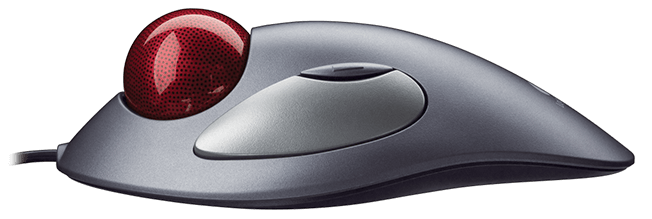I'm leaving some code to handle a simple trackball. The code actually wasn't done by me, but I can't remember where I took it! Originally it was C code which I've wrapped up in a C++ class with way more comments and an example of how to use it. [C++ trackball code ].

# Compute Harmonic weights on a triangular meshHere I describe the discreet Laplace-Beltrami operator for a triangle mesh and how to derive the Laplacian matrix for that mesh. Then I provide [C++ code ] to compute harmonic weights over a triangular mesh by solving the Laplace equation.

# Building a new culture of teaching and learningTheories around alternative systems for teaching and learning has always interested me, so I decided to open an entry about that.  My first contribution will be the English transcript of an inspiring video about education. I might put more articles/videos on this topic later.

# Recipe for implicit surface reconstruction with HRBF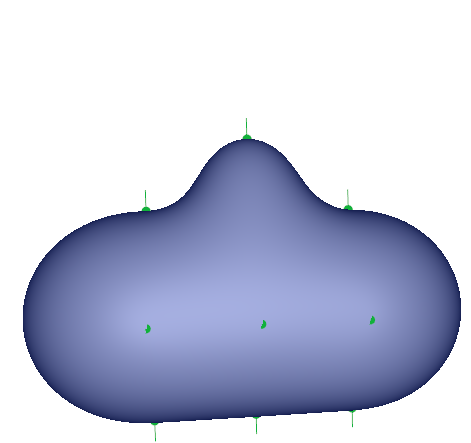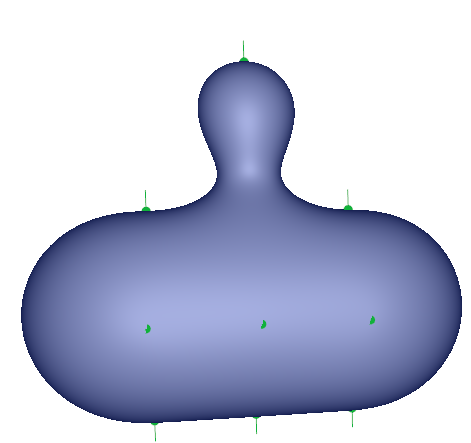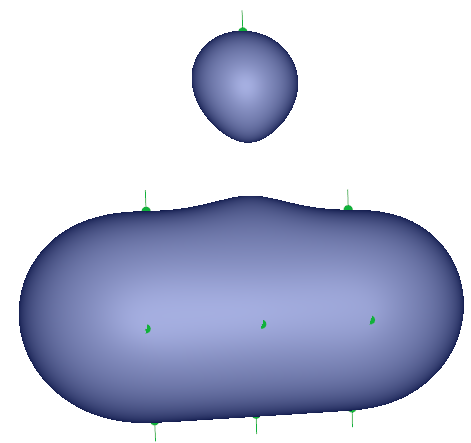In this entry I'll explain and give code for the easiest method I know to reconstruct a surface represented by a scalar field $$f: \mathbb R^3 \rightarrow \mathbb R$$ by interpolating a set of point with their normals. The method is called Hermite Radial Basis Function (HRBF) interpolation. The audience aimed is anyone with some basic knowledge about implicit surfaces (which people often know as metaballs and render with the marching cube algorithm)

•HRBF core sources ] [HRBF toy app sources ] [HRBF toy app binaries
•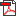maths details ]  [maths summary ] [tex sources (FR) (EN) ]

# C++ code to emulate openGL old direct mode drawing

glBegin(GL_TRIANGLES);
glVertex3f(1.0f, 0.0f, 0.0f);
glVertex3f(0.0f, 1.0f, 0.0f);
glVertex3f(0.0f, 0.0f, 1.0f);
glEnd();


[compact version ] | [modular version ]

Remember the old days when you were able to simply draw a few primitives with GL_POINTS, GL_LINES or GL_QUADS within a pair of good old begin() end(). Well I'm providing a C++ class which will enable you to do this again under OpenGL 3.1 or higher.

# C code for 4x4 matrix inversion

Just leaving some code here to invert either column or row major 4x4 matrices.

# Upgrade to CUDA 5.0: cudaMemcpyToSymbol invalid device symbol error

You have just upgraded to CUDA 5.0 and the function cudaMemcpyToSymbol() throws you the infamous "invalid device symbol" error. Here is what to do.

# CUDA constant memory, namespace, and weird bugs

Edit: the usage of cudaMemcpyToSymbol describded below is deprecated since CUDA 4.1 (See also my new entry Upgrade to CUDA 5.0: cudaMemcpyToSymbol invalid device symbol error)

Today I want to discuss some issues I had with CUDA constant memory and share some workarounds.

# Drag and drop not working in QTDesigner or QTCreator designer

This has upset me for some time and I finally found a forum entry solving this problem.

The problem: I wasn't able to drag&drop in QTDesigner any widgets from the widgets list to the form I was editing.
Solution found here: qtforum.org

You only need to add this entry to your /etc/X11/xorg.conf:

Section "Module"    Load "extmod"EndSection

# Broken F4 shortcut in Qtcreator for cuda files ".cu"

Well I'm using QtCreator to code my CUDA project and there has been a lot of things bothering me. Among them is this F4 shortcut which doesn't work. The shortcut enables switching between the header and source file but apparently the extension .cu is not recognized as a C++ source file. Here is how to fix it.

• 1